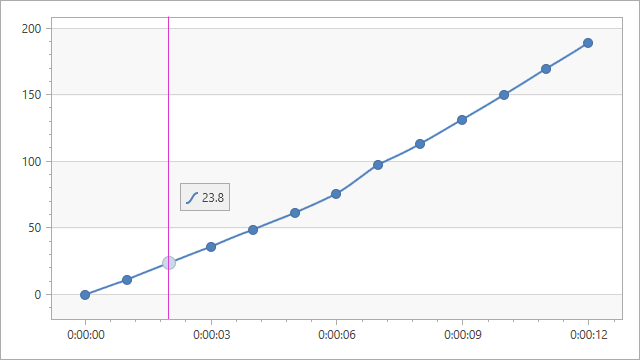# ChartCalculatedFieldType Enum

Lists a calculated field’s returned value types.

Namespace: DevExpress.Xpf.Charts

Assembly: DevExpress.Xpf.Charts.v23.1.dll

NuGet Package: DevExpress.Wpf.Charts

## Declaration

``public enum ChartCalculatedFieldType``

## Members

Name Description
`None`

Indicates that the field’s type is undefined and determined based on the returned object during chart initialization.

`String`

Indicates that the field returns a string value as a sequence of UTF-16 code units (the String type).

`DateTime`

Indicates that the field returns a value expressed as a date and time of day (the DateTime type).

`TimeSpan`

Indicates that the field returns a value as a time interval (the TimeSpan type).

`Byte`

Indicates that the field returns an 8-bit unsigned integer value (the Byte type).

`Int16`

Indicates that the field returns a 16-bit signed integer value (the Int16 type).

`Int32`

Indicates that the field returns a 32-bit signed integer value (the Int32 type).

`Float`

Indicates that the field returns a single-precision floating-point value (the Single type).

`Double`

Indicates that the field returns a double-precision floating-point value (the Double type).

`Decimal`

Indicates that the field returns a decimal value (the Decimal type).

`Boolean`

Indicates that the field returns a Boolean (true or false) value (the Boolean type).

`Guid`

Indicates that the field returns a global unique identifier value (the Guid type).

## Related API Members

The following properties accept/return ChartCalculatedFieldType values:

## Example

The following example shows how to create a calculated field and then use this field as a data source for a series. The field’s values are calculated by the following expression: [Time.Seconds] * [Velocity].``````<dxc:ChartControl x:Name="chartControl">
<dxc:ChartControl.DataContext>
<local:ChartViewModel/>
</dxc:ChartControl.DataContext>
<dxc:ChartControl.CalculatedFields>
<dxc:ChartCalculatedField FieldName="Displacement"
Expression="[Time.Seconds] * [Velocity]"
FieldType="Double"/>
</dxc:ChartControl.CalculatedFields>
<dxc:XYDiagram2D>
<dxc:SplineSeries2D DisplayName="Series"
DataSource="{Binding DataPoints}"
ArgumentDataMember="Time"
ValueDataMember="Displacement"
CrosshairLabelPattern="{}{Displacement:f1}"
MarkerVisible="True"/>
...
</dxc:XYDiagram2D>
</dxc:ChartControl>
``````